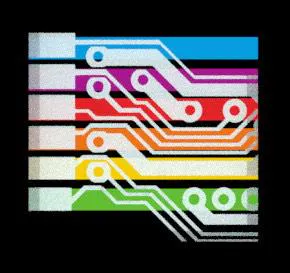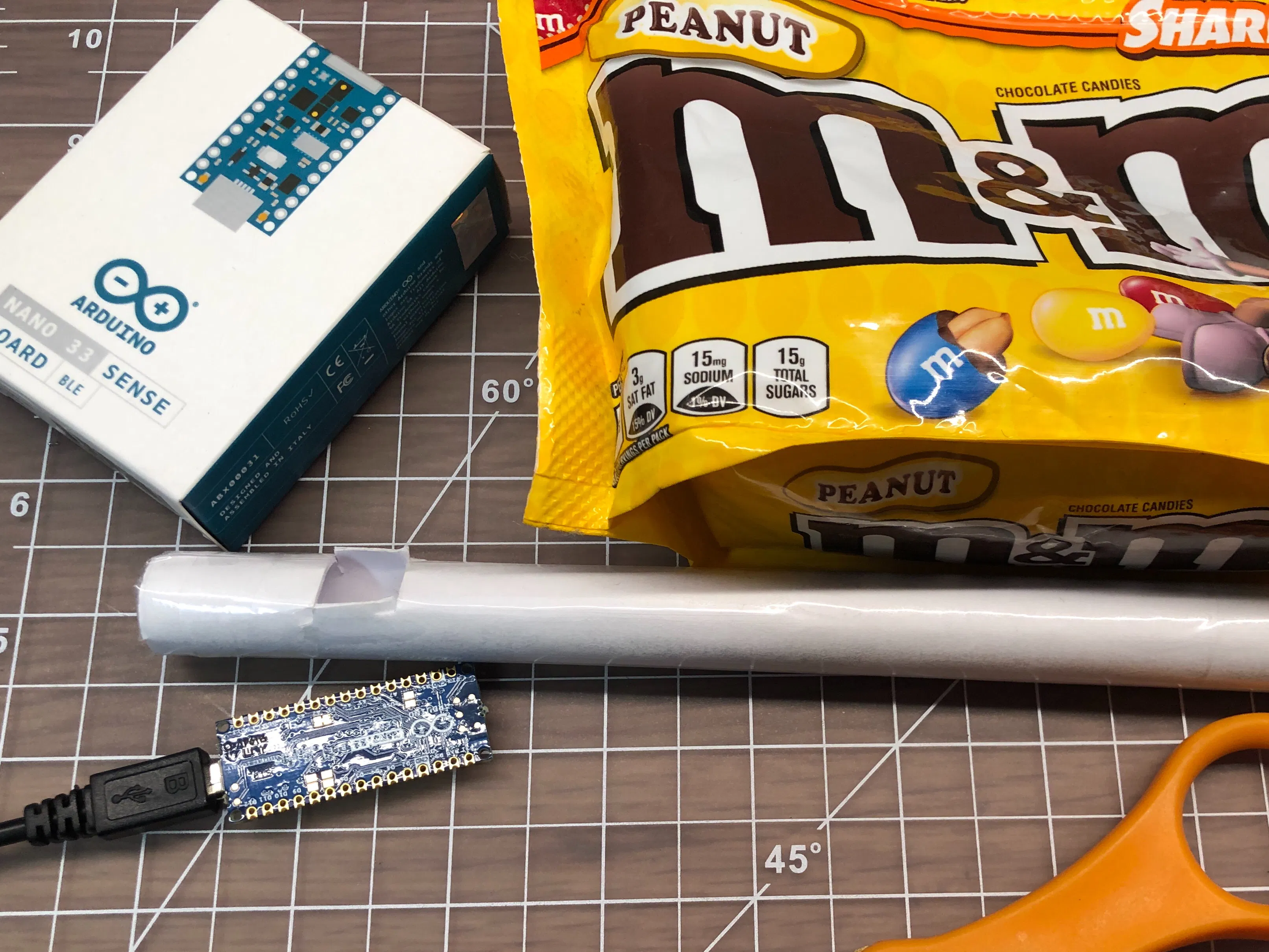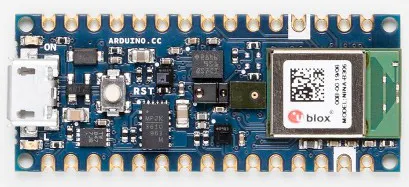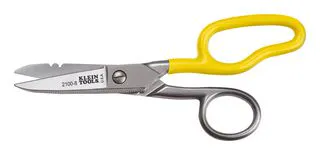# Classify Candy in Free Fall Using TinyML

Using the Arduino KNN library to classify the color of M&Ms we throw at it.

IntermediateShowcase (no instructions)1,744## Things used in this project

### Hardware componentsArduino Nano 33 BLE Sense
×1

### Hand tools and fabrication machinesScissors, Free Fall

## Code

### Arduino sketch

Arduino
Classify object without using proximity, using illumination from the Arduino onboard LEDs
```/*

k-NN color classification
-------------------------

This sketch classifies objects using a color sensor.

First you 'teach' the Arduino by putting an example of each object close to the color sensor.
After this the Arduino will guess the name of objects it is shown based on how similar
the color is to the examples it has seen.

This example uses a simple case of k-Nearest Neighbour (k-NN) algorithm where k=1.

HARDWARE: Arduino Nano BLE Sense

USAGE: Follow prompts in serial console. Move object close to the board to sample its color, then move it away.

Works best in a well lit area with objects of different colors.

NOTE: Make sure Serial Monitor's line ending setting is configured for "Newline" or "Both NL & CR".

This example code is in the public domain.

*/

#include <Arduino_KNN.h>
#include <Arduino_APDS9960.h>

const int INPUTS = 3; // Classifier input is color sensor data; red, green and blue levels
const int CLASSES = 3; // Number of objects we will classify (e.g. Apple, Banana, Orange)
const int EXAMPLES_PER_CLASS = 1; // Number of times user needs to show examples for each object

// K=1 means the classifier looks for the single closest color example it's seen previously
const int K = 1;

// Create a new KNNClassifier
KNNClassifier myKNN(INPUTS);

// Names for each class (object type)
String label[CLASSES];

// Array to store data to pass to the KNN library
float color[INPUTS];

// Threshold for color brightness
const float THRESHOLD = 0.98;
int initialAmbient = 0;

void setup() {

Serial.begin(9600);
while (!Serial);

// Pins for the built-in RGB LEDs on the Arduino Nano 33 BLE Sense
pinMode(LEDR, OUTPUT);
pinMode(LEDG, OUTPUT);
pinMode(LEDB, OUTPUT);

if (!APDS.begin()) {
Serial.println("Failled to initialized APDS!");
while (1);
}

Serial.println("Arduino k-NN color classifier");

// Ask user for the name of each object
for (int currentClass = 0; currentClass < CLASSES; currentClass++) {

Serial.println("Enter an object name:");

// Ask user to show examples of each object
for (int currentExample = 0; currentExample < EXAMPLES_PER_CLASS; currentExample++) {

Serial.print("Show me an example ");
Serial.println(label[currentClass]);

// Wait for an object then read its color

// Add example color to the k-NN model

}
}
}

void loop() {

int classification;

// Wait for the object to move away again
while (!APDS.proximityAvailable() || APDS.readProximity() == 0) {}

// Wait for an object then read its color

// Classify the object
classification = myKNN.classify(color, K);

// Print the classification
Serial.println(label[classification]);
Serial.println();

}

int red, green, blue, ambient;
while (!APDS.colorAvailable()) {};
return ambient;
}

int red, green, blue, ambient = 0, colorTotal = 0;

// Wait until shadow of object passing
while (readAmbient() > initialAmbient * THRESHOLD) {}

// Wait until we have a color bright enough
while (ambient < initialAmbient * THRESHOLD) {

// Sample if color is available and object is close
if (APDS.colorAvailable()) {

colorTotal = (red + green + blue);
}
}

// Normalise the color sample data and put it in the classifier input array
color = (float)red / colorTotal;
color = (float)green / colorTotal;
color = (float)blue / colorTotal;

// Print the red, green and blue percentage values
Serial.print(color);
Serial.print(",");
Serial.print(color);
Serial.print(",");
Serial.println(color);
}

// reads a name from the Serial Monitor
String line;

while (1) {
if (Serial.available()) {

if (c == '\r') {
// ignore
continue;
} else if (c == '\n') {
break;
}

line += c;
}
}

return line;
}
```

## Credits

### 8bitkick

3 projects • 25 followers